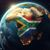# using the field calculator with python

3723
3
07-27-2016 11:41 AMNew Contributor II

Hello,

So i am trying to use the field calculator using python to determine the input for the field. So for example my table has 2 fields(both text fields(one of the fields would be empty at this point)), i would like the code to say If field one is "String 1" then put in field 2 "string 2". Basically i want it to check the value of field one and if it matches the string i am looking for then i want it to write another string that i decide in the code to write. I was wondering if  someone had a template of a similar code that i can look at to get an idea on how to write it. Preferable in python but i think VB works also. Thanks in advance

3 RepliesbyMVP Legendary Contributor

the best bet would be to either show the code you are trying to implement or show the field calculator syntax you are using since your description is a bit of bothbyMVP Honored Contributor

See the third example on this page, which uses if-then logic in a code block: Calculate Field—Help | ArcGIS for DesktopbyMVP Regular Contributor

So you basically want to calculate Field 2 based on the value in Field 1

Your Python code block can look like this:

``````def calcfield(field1):
val = ""
if field1 == "test":
val = "string 2"
else:
val = "other string"
return val‍‍‍‍‍‍‍‍‍‍‍‍‍‍‍‍‍‍‍‍‍``````

And the field calculation expression:

Field2 =

``calcfield(!MyField!)‍``